• 对符号表达式或函数表达式赋值
千次阅读
2017-02-09 11:53:32
syms x y;%定义变量x，y
z=x^5*y^3;%表达式
x1=[0:20];
y1=[0:20];
z1=subs(z,{x,y},{x1,y1});%可使用x1,y1,z1绘制三维图
disp(z);%写出表达式函数
更多相关内容
• 但是经过运算完成后有时候是需要把运算得到的结果转化回矩阵进行后续的操作的，这个时候就需要通过一个矩阵对符号函数赋值从而达到转化出来一个新的矩阵。 subs 这个函数常用的情况下有三个参...

matlab里面的符号函数通常指的是用syms创建出来一个变量，然后对这些变量进行的运算。比如：

syms x
y=x.^2

matlab可以比较方便地对一个符号函数求导或者积分，傅里叶变换拉普拉斯变换等。但是经过运算完成后有时候是需要把运算得到的结果转化回矩阵进行后续的操作的，这个时候就需要通过一个矩阵对符号函数赋值从而达到转化出来一个新的矩阵。

subs

这个函数常用的情况下有三个参数，用比较通俗的数学语言来说，就是函数、自变量、一个数字（你要把哪个数字赋值给这个符号函数）。

当然除了数字还可以是字符，如果格式匹配的话，上面只是举个例子。

具体调用的时候就是像下面这样

y1=subs(y,x,10);

这就是将10赋值给符号函数的一种情形，同样也可以用矩阵进行赋值，这样就可以还原出这个符号函数对应的矩阵。

t=0:0.01:10;
y1=subs(y,x,t);

inline

这个函数据说是可以使用，不过在matlab 2017a的版本上调用的时候出现了下面的情况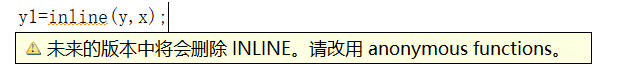它说未来会删除，但是毕竟现在还是没有删除的嘛，那么就强行调用一下……

结果……结果……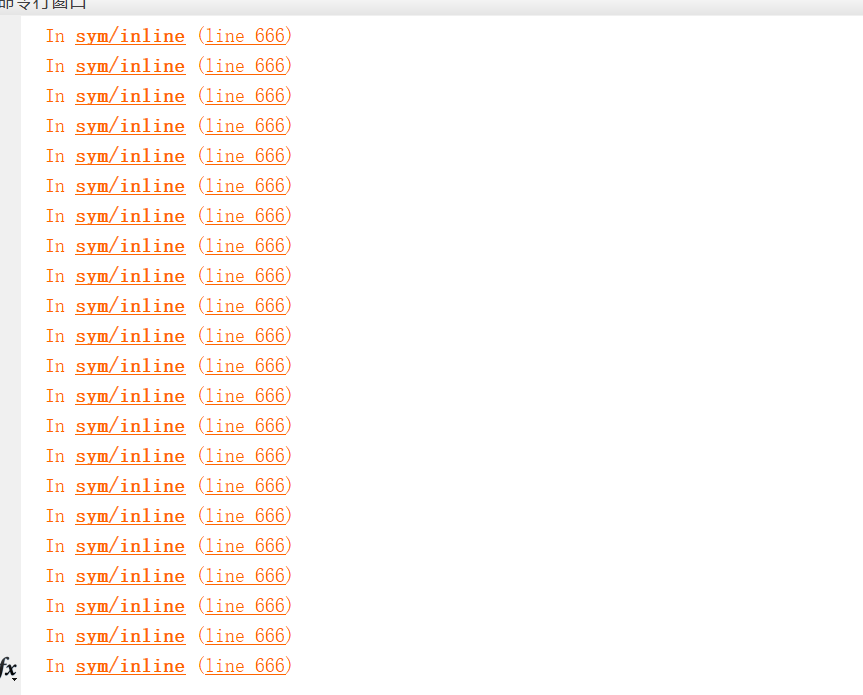命令行窗口打印出了无数的东西……没错，，一包辣条都吃完了程序居然还没有跑完，就这么一句。所以只好点上面的停止调试按钮……路过的大神万望指点，或者matlab2017以上真的已经不能用了。

@

@不是一个函数，而是matlab里面的一种操作，叫做定义匿名函数。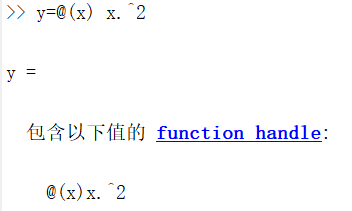命令行里面写下这样的函数会看到这样的结果，嗯，这是一个函数的句柄。

调用的时候就像这样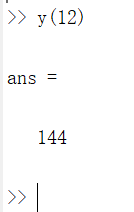这给人一种怎样的感觉？我们平时辛辛苦苦写好的函数通常都要生成.m文件，留着以后使用，毕竟只使用一次的函数略微淡化了函数存在的价值。

但是这个函数呢，这个函数是一个句柄，定义好了之后它是当前变量空间里面的一个变量，可以通过圆括号给它赋值获得回传的结果。然后，当matlab关闭了之后，这样定义好了的一个变量就不再存在了。

这样看起来，平常使用的函数像是家庭保姆，而匿名函数像是临时找来的保洁阿姨。但是匿名函数有它本身的优点，最显而易见的就是方便。其它优点以后补充。。。

然而，，它并不能解决在开头提到的那个问题。比如定义了一个这样的匿名函数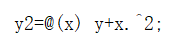其中y就是上面定义过的y。然后调用匿名函数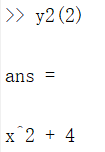这印证了我们对于匿名函数的误会。它内部的函数结构是需要在定义函数的时候当场指定的，里面的x指的就是赤裸裸的后面的x，y里面蕴含的x它是不会管的。所以我们最开始那种思路，先对符号函数进行某些运算，最后再通过匿名函数转换，这种思路是行不通的。

所以，最后似乎只有subs可以解决问题，不过匿名函数的潜在功能更强大，只不过在这个问题上并不如subs优越

展开全文• 符号表是一种用于语言翻译器（例如编译器和解释器）中的数据结构。在符号表中，程序源代码中的每个标识符都和它的声明或使用信息绑定在一起，比如其数据类型、作用域以及内存地址。 符号表在编译程序工作的过程中...

符号表是一种用于语言翻译器（例如编译器解释器）中的数据结构。在符号表中，程序源代码中的每个标识符都和它的声明或使用信息绑定在一起，比如其数据类型、作用域以及内存地址

符号表在编译程序工作的过程中需要不断收集、记录和使用源程序中一些语法符号的类型和特征等相关信息。这些信息一般以表格形式存储于系统中。如常数表、变量名表、数组名表、过程名表、标号表等等，统称为符号表。对于符号表组织、构造和管理方法的好坏会直接影响编译系统的运行效率。

（上文来自百度词条）

符号表的属性：1.符号名 2.符号的类型 3.符号的存储类别  4.符号的作用域及可视性 5.符号变量的存储分配信息

6.符号的其它属性 （1）数组内情向量 （2）记录结构型的成员信息（3） 函数及过程的形参

编译程序按名字的不同种属分别使用许多符号表，如常熟表。变量名表 过程名表 函数入口名表

符号表的关键字域（段）就是符号名称等长关键字域（段）符号表

不等长关键字段符号表---采用关键字词的索引结构。

关键字在符号表的查找中相当重要 hash函数构造方法就是以取关键字的值不同区分

如 直接定址伐 数字分析法 折叠法

变量名是给编译器看的，编译器根据变量是局部还是全局分配内存地址或栈空间，所谓的变量名在内存中不存在，操作时转换成地址数存放在寄存器中了。其实可以理解为是符号表起到了连接作用。

展开全文• MATLAB中可以使用命令diff对符号函数进行求导，并且当diff作用到向量或者是矩阵时还可以求差分 1、符号函数求导 1.1 一元符号函数求导 先定义一元符号函数 syms x y = x^4-5*x^2+6 进行求导，当diff作用到符号函数...

声明：本文章中数据来自清风老师数学建模课程

# MATLAB符号函数的求导

MATLAB中可以使用命令diff对符号函数进行求导，并且当diff作用到向量或者是矩阵时还可以求差分

## 1、符号函数求导

### 1.1 一元符号函数求导

先定义一元符号函数

syms x
y = x^4-5*x^2+6


进行求导，当diff作用到符号函数上时可以对符号函数进行求导

• 第一个参数传入符号函数
• 第二个参数传入要求几阶导，第二个参数空着默认求1一阶导
diff(y,1) %求一阶导数  diff(y)默认求一阶导
% 4*x^3 - 10*x
diff(y,2) %求二阶导数
% 12*x^2 - 10


求导后分析结果遇到的问题
下面我们用diff对cos(x)*tan(x)进行求导

syms x
y = cos(x)*tan(x)
dy = diff(y,10)  %求十阶导数

240*sin(x)*(tan(x)^2 + 1)^2 - cos(x)*tan(x) - 4032*sin(x)*(tan(x)^2 + 1)^3 + 32640*sin(x)*(tan(x)^2 + 1)^4 - 79360*sin(x)*(tan(x)^2 + 1)^5 - 10*sin(x)*(tan(x)^2 + 1) - 3360*cos(x)*tan(x)*(tan(x)^2 + 1)^2 + 57120*cos(x)*tan(x)*(tan(x)^2 + 1)^3 - 1680*cos(x)*tan(x)^3*(tan(x)^2 + 1) - 357120*cos(x)*tan(x)*(tan(x)^2 + 1)^4 + 353792*cos(x)*tan(x)*(tan(x)^2 + 1)^5 + 6720*cos(x)*tan(x)^5*(tan(x)^2 + 1) - 5760*cos(x)*tan(x)^7*(tan(x)^2 + 1) + 512*cos(x)*tan(x)^9*(tan(x)^2 + 1) + 480*sin(x)*tan(x)^2*(tan(x)^2 + 1) - 4032*sin(x)*tan(x)^4*(tan(x)^2 + 1) + 7680*sin(x)*tan(x)^6*(tan(x)^2 + 1) - 2560*sin(x)*tan(x)^8*(tan(x)^2 + 1) + 87360*cos(x)*tan(x)^3*(tan(x)^2 + 1)^2 - 1105920*cos(x)*tan(x)^3*(tan(x)^2 + 1)^3 + 1841152*cos(x)*tan(x)^3*(tan(x)^2 + 1)^4 - 345600*cos(x)*tan(x)^5*(tan(x)^2 + 1)^2 + 1304832*cos(x)*tan(x)^5*(tan(x)^2 + 1)^3 + 128512*cos(x)*tan(x)^7*(tan(x)^2 + 1)^2 - 22176*sin(x)*tan(x)^2*(tan(x)^2 + 1)^2 + 345600*sin(x)*tan(x)^2*(tan(x)^2 + 1)^3 - 1372160*sin(x)*tan(x)^2*(tan(x)^2 + 1)^4 + 218880*sin(x)*tan(x)^4*(tan(x)^2 + 1)^2 - 1858560*sin(x)*tan(x)^4*(tan(x)^2 + 1)^3 - 316160*sin(x)*tan(x)^6*(tan(x)^2 + 1)^2 + 90*cos(x)*tan(x)*(tan(x)^2 + 1)


然而并不理想，见下图：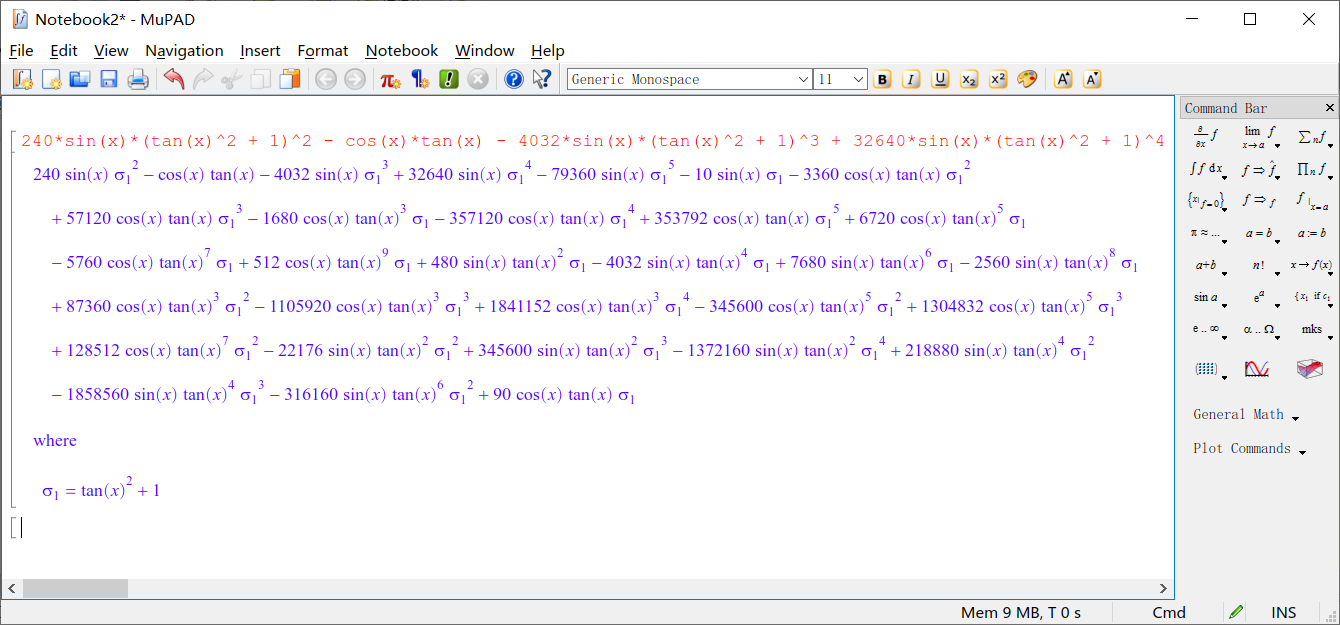这时候我们可以尝试一下simplify来进行化简整理

如下图所示，结果很明显，是我们想要的形式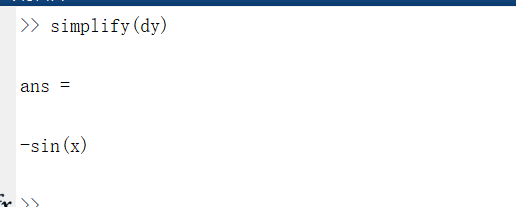那么为什么我们使用diff对cos(x)*tan(x)求导的结果这么复杂呢，其实它的求导算法就是莱布尼兹公式的展开。其实我们知道cos(x)*tan(x)等于sin(x),求高次导数一定是一个简单的式子

### 1.2 多元函数的导数

参数：

• 第一个参数是待求导符号函数
• 第二个参数是要对那个自变量求偏导
• 第三个参数是要求几阶偏导数

当连续对自变量求偏导时，放在前面的自变量先求偏导，后面的自变量后求偏导

syms x1 x2 x3
y1 = x1^5*x2+x2*x3-x1^2*x3
py1 = diff(y1,x1,1) % 对x1求一阶偏导
% 5*x2*x1^4 - 2*x3*x1
py2 = diff(y1,x1,2) % 对x1求二阶偏导
% 20*x2*x1^3 - 2*x3
py3 = diff(y1,x1,x2) % 先对x1求偏导，再对x2求偏导
% 5*x1^4
py4 = diff(y1,x2,x1) % 先对x2求偏导，再对x1求偏导
% 5*x1^4


## 2、差分

注意，如果diff函数作用的对象不是符号函数，而是矩阵，那么对应的功能是求差分。

### 2.1 向量的差分

默认求1阶差分

A=[4 5 6 3 2 1];
diff(A)  % 求向量A的一阶差分     1     1    -3    -1    -1
diff(A,2)  % 在一阶差分的基础上再差分一次     0    -4     2     0


### 2.2 矩阵的差分

默认是列的方向上求差分（下一行减上一行），且默认求一阶差分。
第三个参数指定为2时，在行的方向上求差分（后一列减前一列）
第二个参数用于指定求几阶差分，默认一阶差分

A=[4 5 6;
7 4 2;
5 6 2]
A1=diff(A)  % 下一行减去上一行求一阶差分
%      3    -1    -4
%     -2     2     0
A2=diff(A,2) % 下一行减去上一行求二阶差分（在一阶差分的基础上再差分一次）
%     -5     3     4
A3=diff(A,2,1) % 最后面的1表示在行上进行差分（在列的方向上进行差分）
%     -5     3     4
A4=diff(A,1,2)  % 后一列减去前一列求一阶差分， 最后面的2表示在列上进行差分（在行的方向上进行差分）
%      1     1
%     -3    -2
%      1    -4
A4=diff(A,2,2) % 后一列减去前一列求二阶差分
%      0
%      1
%     -5


展开全文matlab
• 材料/工具：word2010在菜单栏中打开“插入”菜单栏在“插入”菜单栏中找到“符号”，点击进入“插入符号”栏在“插入符号”栏中点击“其他符号”从字体搜索框找到“Wingdings 2”。excel中怎么输入对号和错号？输入...
• horner函数实现对符号表达式进行嵌套 R = horner(P)：P为待嵌套的符号表达式，R为嵌套后的符号表达式 &gt;&gt; syms x y &gt;&gt; horner(x^3 - 6*x^2 + 11*x - 6) ans = x*(x*(x - 6) + 11) ...matlab 基础 笔记
• 本实验源于中南大学《科学计算与MATLAB语言...将由A来建立符号对象，其中，A可以是一个数值常量、数值矩阵或数值表达式(不加单引号),此时符号对象为一个符号常量；A也可以是一个变量名(加单引号),这时符号对象为一个...
• ## PTA 符号配对

千次阅读 2019-02-02 00:26:19
符号配对 （20 分） 请编写程序检查C语言源程序中下列符号是否配对：/与/、(与)、[与]、{与}。 输入格式: 输入为一个C语言源程序。当读到某一行中只有一个句点.和一个回车的时候，标志着输入结束。程序中需要检查...
• 输入和输出都是二进制补码，补码的符号位扩展就是补最高位即符号位即可。 三. 模块代码 /* * @Author : Xu Dakang * @Email : XudaKang_up@qq.com * @Date : 2021-05-14 09:50:48 * @LastEditors : Xu Daka.补码 二进制 Verilog verilog
• 一、符号表达式的极限 limit(F,x,a)：求当时，符号表达式F的极限。 limit(F,a)：符号表达式F采用默认自变量（可由函数findsym求得），该函数求F的自变量趋于a时的极限值。 limit(F)：符号表达式采用默认变量，并...
• 弄个好玩的,怎么吧vscode 的符号设置为组合形式,如下: 看这位大佬的github:https://github.com/tonsky/FiraCode 安装字体地址 配置方法 安装完字体后,打开vscode,配置如下 完成…搞起来vscode
• MATLAB符号运算处理的主要对象是符号符号表达式，为此要使用一种新的数据类型---符号变量。符号表达式的创建可由符号''或sym函数来完成。matlab
• 提示：文章写完后，目录可以自动生成，如何生成可参考右边的帮助文档 文章目录0. 前言1. SAX的特性2. SAX算法2.1 表示方法2.1...表示的独特之处在于它允许降维，它还允许在符号表示上定义距离度量，该符号表示下界对应算法 数据库 大数据
• 在C语言中符号常量是由宏定义“#define”来定义的常量,以下叙述中正确的是()在下列的符号常量定义中，正确的定义格式为()A.#defineM1B.constintM220C.#defineM3定义符号常量通常使用宏定义命令关键字()下面叙述错误...
• ## 符号表的作用

千次阅读 2020-03-31 20:57:31
在编译程序中符号表用来存放语言程序中出现的有关标识符的属性信息，这些信息集中反映了标识符的语义特征属性。在词法分析及语法在分析过程中不断积累和更新表中的信息，并在词法分析到代码生成的各阶段，按各自的...
• https://jingyan.baidu.com/article/7082dc1cdbd69de40a89bdf8.html http://blog.sina.com.cn/s/blog_7f15852e0101g8tq.html
• 对符号表的操作: 填入名称. 查找名字. 访问信息. 填写修改信息. 删除. 对符号表进行操作的时机: 定义性出现. 使用性出现. 按名字的不同种属建立多张符号表,如常数表,变量名表,过程名表,… 符号的组织方式:...
• 1、图层右键属性，符号系统，类别，唯一值，值字段选择类型字段，增加值，点击值，点击增加。也可以增加新值。 2、双击符号，为每一种符号设置样式。（其他所有值去掉勾选）arcgis
• 符号积分 int(f,a,b) %f求定积分，下上限为a,b int(f) %f求不定积分 int(f,'t') %用t作为变量求f的不定积分 syms x %定义x为变量 y=x.^3+2*x.^2+2; %创建函数y=x^3+2*x^2+2 q=int(y,1,2) %用int...
• 对于无符号数以及有符号数值左移效果一致，如： //默认i为32位 int i = 1; //i = 0000 0000 0000 0000 0000 0000 0000 0001 i = i << 31;//i = 1000 0000 0000 0000 0000 0000 0000 0000, i = -2147483648 ...
• ## 符号配对

千次阅读 2018-09-28 11:45:03
7-2 符号配对 （20 分） 请编写程序检查C语言源程序中下列符号是否配对：/*与*/、(与)、[与]、{与}。 输入格式: 输入为一个C语言源程序。当读到某一行中只有一个句点.和一个回车的时候，标志着输入结束。程序中...
• 1 在目标代码生成阶段，符号表用D A．目标代码生成 B．语义检查 C． 语法检查 D．... 2 在编译处理过程中,符号表只有在词法分析...C 给定名字，往表中填写或更新它的某些信息 D 删除一个或一组无用的项 4 目...
• R=subs(S)：用工作区中的变量值替换符号表达式中的某一特定符号。 R=subs(S,New)：用新符号变量New来替换符号表达式S中的默认变量。 R=subs(S,Old,New) &gt;&gt; syms x y &gt;&gt; f=sym('x^2+x...
• 第八章复习题 在目标代码生成阶段，符号表用（D）。 A．目标代码生成 B．语义检查 C．语法检查 D．...在编译处理过程中,符号表只有在词法分析阶段和语法分析阶段才是有用。...目标代码生成阶段，对符号...编译原理
• 答：可以用：subs(符号变量/符号变量的矩阵) syms z1 z2 z3 z4 z11 z22 z33 z44 IL1 IL2 Us1 Us2 A=[1,-1,0,0,0,0,0,0;  0,1,1,0,0,0,0,0;  0,0,0,0,1,-1,0,0;  0,0,0,0,0,1,1,0;  1,0,0,1,1,0,0,1;  z1,z2,-...
• ## C++有符号和无符号之间的转换

千次阅读 多人点赞 2021-06-04 18:51:49
先来看一个程序： #include int main() { ...-20正常打印我们都知道，但当一个有符号和一个无符号之间的数进行相加减会发生什么呢？ 是这样的：a+b，首先把负数转换为无符号数，然后在进行运算。 b
• 名字完全一样，而且都是GLOBAL的 GLOBAL的符号即全局的符号，同名的全局符号会被认为是同一个符号，由于main先加载了libA，得到了libA中的subfunc符号，再加载libB时，就把libB中的subfunc忽略了。 解决方案 这其实...
• 量词符号 任意：∀ 存在：∃ 且：∧ 或：∨ 非：﹁ 数学符号 ≈ ≡ ≠ ＝ ≤≥ ＜ ＞ ≮ ≯ ∷ ± ＋ － × ÷ ／ ∫ ∮ ∝ ∞ ∧ ∨ ∑ ∏ ∪ ∩ ∈ ∵ ∴ ⊥ ‖ ∠ ⌒ ≌ ∽ √ （） 【】｛｝ Ⅰ Ⅱ ⊕ ⊙∥α ...
• 一、符号计算 1、符号计算特点  1、计算精确：符号计算基于数学公式、定理并通过一系列推理、演绎得到方程的解或者数学表达式的值... 3、对待符号计算态度：用其来完成公式推导和解决简单的计算时效性要求不高...
• ## 11. 符号和符号解析

千次阅读 多人点赞 2018-12-04 21:35:00
11.1 符号符号表 1. 符号的定义和引用 局部变量temp分配在栈中，不会在过程外被引用，因此不是符号定义 2. 连接符号的类型 （1）三种类型 每个可重定位目标模块m都有一个符号表，它包含了在m中定义和引用的符号,......